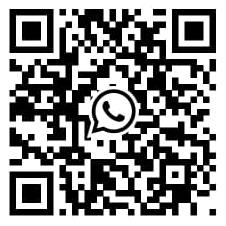# Association test

Hypothesis tests are widely used to assess the association between two or more variables. To find out if two variables are linked in some way, an association test is performed. The measure of association refers to the bivariate correlation and regression coefficients that measure the strength and direction of the relationship. A bivariate association test includes one independent variable and one dependant variable while a Multivariate association test includes more than two variables. This is closely tied with homework help on correlation analysis which is a type of association test. Among the association, tests are parametric and nonparametric tests depending on the applicability of the methods, with the question of comparing variables that are non-stochastic or continuous being of particular interest in modern studies. Assignment help may involve a wide array of tests that calculate association by comparing ratios, such as the chi-square. This test is therefore also known as the Chi-square test.

## To measure the statistical association, researchers need to understand the following point:-

1. There is a difference between measures of association and measures of statistical significance
2. In a measure of association, the value of zero represents no relationship. In correlation analysis(r), the value of one represents a perfect relationship, the same is for standardised beta weight, which signifies a perfect relationship for the value one.

## Test Vs. Measure of Association

There is a subtle difference between these two, test association and measure of association. Measure association quantifies the relationship while test association assigns statistical significance to your results. Also, a test is more rigorous than a measure.

## Common Tests for Association

1. The chi-square test is used to find a relationship between two categorical variables for the association.
2. The Cochran-Mantel-Haenszel (CMH) Test studies data from various sources.
3. Fisher’s Exact Test works like a chi-square test when you have a small sample size.
4. The gamma coefficient signifies how strong the relationship is in between two pairs of data points “match”.
5. Goodman Kruska’s Gamma test is performed for ranked variables.

## Common Measures of Association

### Pearson’s correlation coefficient

Pearson’s correlation coefficient, r (rho), measures the strength on a continuous scale for the linear relationship between two variables. The coefficient r takes on the values of −1 through +1. The values of these -1 and +1 signify a perfect relationship whereas the value 0 indicates no relationship. Correlation coefficients that differ from 0 but are not −1 or +1 indicate a linear relationship, but not a perfect linear relationship. In practice, the population correlation coefficient(p) is estimated by r, which is the correlation coefficient derived from sample data.

### Spearman rank-order correlation coefficient

To measure the strength of a monotonic association between two variables the Spearman rank-order correlation coefficient (Spearman rho) is designed. These two variables are measured on two variables measured on an ordinal or ranked scale. The result from these two, ordinal and scale are not truly interval in nature.

### Chi-square test

To determine the significant disproportionate membership among groups, an association between gender and political party is done through the chi-square test. This test focus on the measure of the significance of association rather than the strength of association.

### Relative risk and odds ratio

Relative risk measures the strength of an association applied to categorical data derived from an epidemiologic cohort study. The value 1 of the relative risk indicates no association, whereas a relative risk other than 1 indicates an association.

Similarly, an odds ratio is used to measure the strength of association for categorical data derived from a case-control study. The level of strength in the association between risk factors and traits is shown by the odds ratio.

We at SPSS tutor assist you in the whole journey of market research by addressing each of your queries properly so that you would have an appropriate sample size to obtain robust results. From the initial level of methodology and survey development to designing and implementing the analysis plan to the final publication-ready write-up of your results, we stay connected to you with our subject experts.

You can also send us an email   or call us directly on, (+44) 20 3287 0255 and will get in touch with you shortly

I had to submit a big report within a few days. I thought it was impossible to do so. But experts from SPSS Tutor Saved the day.

Paul

had complex research about a medical condition. It was difficult to finish the tasks. But thanks to the SPSS tutor, they helped me to finish tasks on time and made it look easy.

Emily

I had to write an assignment for an IT topic. It required research on new technology. Finding information about it was difficult because it is new. But the SPSS tutor team helped me with it and today I got the highest grades in the class.

Andrew

They helped me with my statistics homework. The team is friendly, the service is nice and I liked how they kept asking me suggestions. I loved their service and would recommend it to others.

Kimberly

Whatsapp Scan